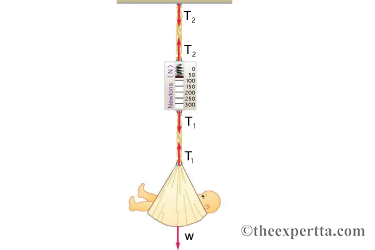# Problem: Consider the baby being weighed in the figure.1. What is the mass (in kg) of the child and basket if a scale reading of 56N is observed?2. What is the tension T1 in the cord attaching the baby to the scale?3. What is the tension T2 in the cord attaching the scale to the ceiling, if the scale has a mass of 0.485 kg?

###### FREE Expert Solution
87% (291 ratings)View Complete Written Solution
###### Problem DetailsConsider the baby being weighed in the figure.

1. What is the mass (in kg) of the child and basket if a scale reading of 56N is observed?

2. What is the tension T1 in the cord attaching the baby to the scale?

3. What is the tension T2 in the cord attaching the scale to the ceiling, if the scale has a mass of 0.485 kg?